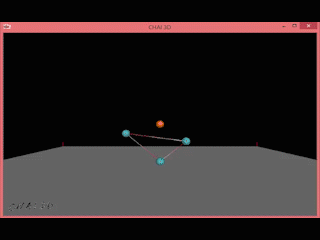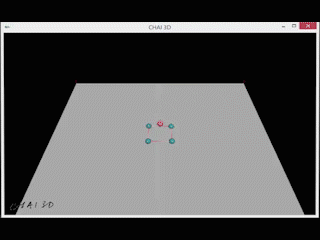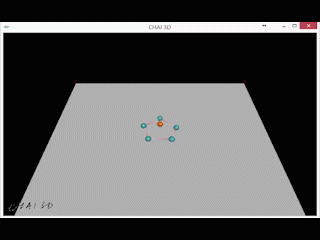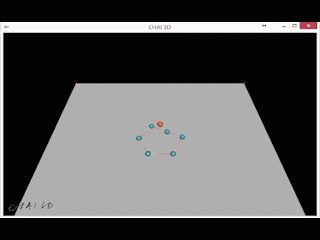# CHAI3D: particle spring system

- 1 min# Techniques

## Spring’s force

The spring force was handled by the formula f = – k * (d’ – d), d was the original length of the spring and d’ was the new length of the spring, f was the force. In the github code, this function was in the Spring.h -> applyForce() function.

## Gravity

The gravity was also handled in this system, line 482 (Main.cpp) & Point.h -> applyAcceleration(cVector3d force) were the code for handling the gravity in this system.

## Collision

The collision between all the particles and the ground. The ground was cMesh object and had the AABBCollisionDetector with two triangles (check the code line 272&294 (Main.cpp)). The collision detection was handled by the computeCollision() function in the line 46 (Point.cpp).

### Collision between particles

Reference link

The collision between all the particles was handled by the formula: d = s1.pos – s2.pos . If d > s1.radius + s2.radius then there is no collision. If d < s1.radius + s2.radius then there is collision between these two points. Check the code blow

bool Point::isCollided(Point *point)
{
// the length between two sphere
double d = cSub(this->point->getPos(), point->point->getPos()).length();
double threshold = radius + point->getRadius();
if (d > threshold)
{
return false;
}
else
{
return true;
}
}

comments powered by Disqus